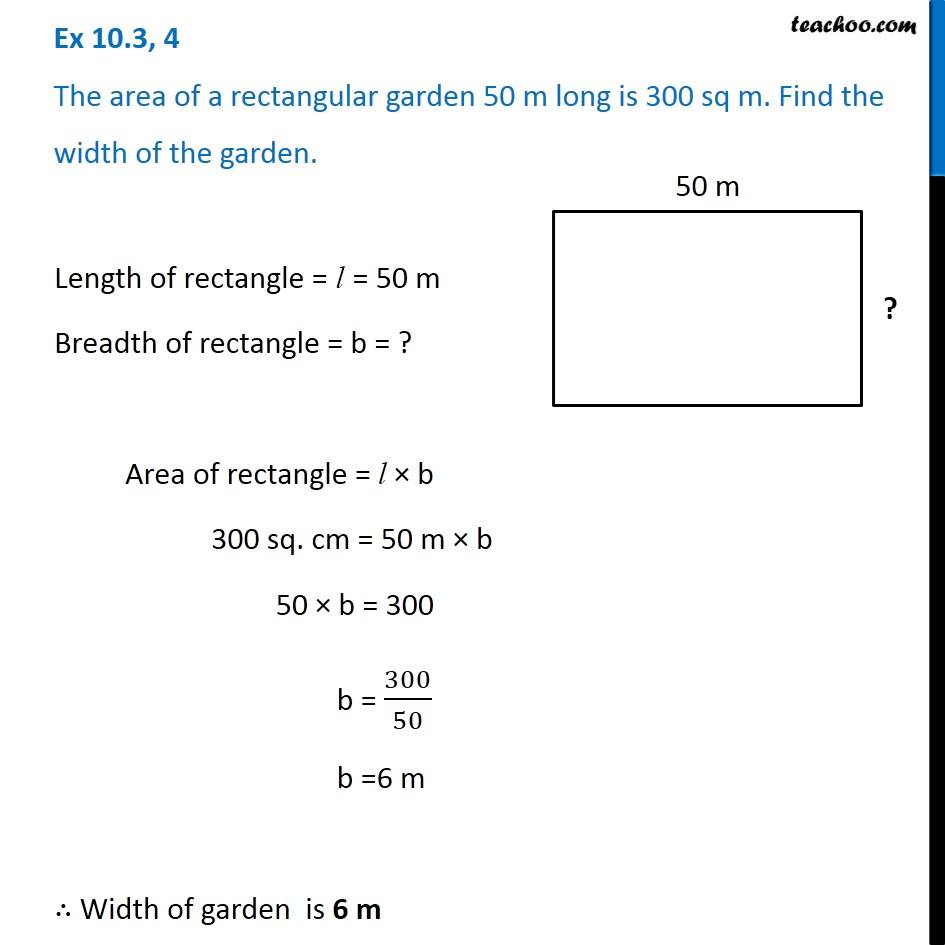Ex 10.3

Chapter 10 Class 6 Mensuration
Serial order wiseLearn in your speed, with individual attention - Teachoo Maths 1-on-1 Class

### Transcript

Ex 10.3, 4 The area of a rectangular garden 50 m long is 300 sq m. Find the width of the garden. Length of rectangle = l = 50 m Breadth of rectangle = b = ? Area of rectangle = l × b 300 sq. cm = 50 m × b 50 × b = 300 b = 300/50 b =6 m ∴ Width of garden is 6 m